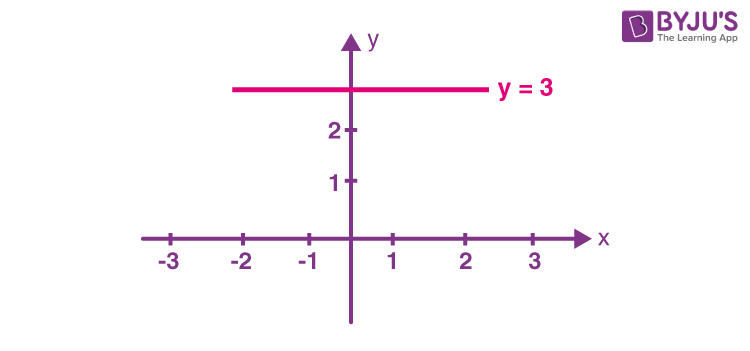# Give the geometric representation of linear equation: y = 3 as in two variables.

We need to give the geometric representation of linear equation: y = 3 as in two variables.

Given,

y = 3

0x + y = 3

0x + y – 3 = 0

Thus, it is a line parallel to the x-axis at a distance of 3 units.(0)(0)